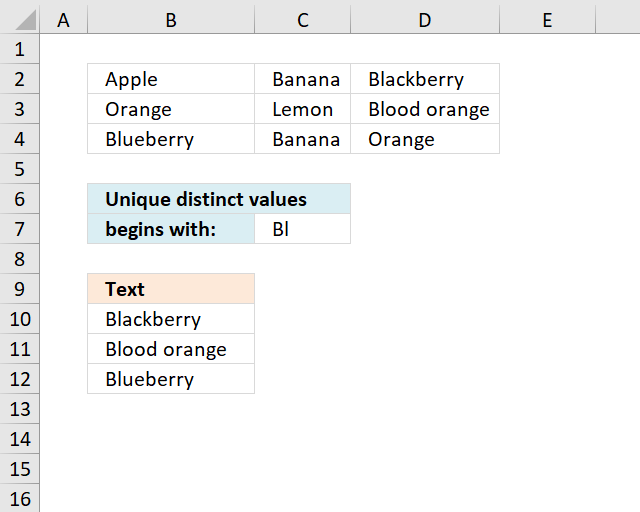Author: Oscar Cronquist Article last updated on August 11, 2019The array formula in cell B10 extracts unique distinct values from cell range B2:D4 that begins with a given condition specified in cell C7.

Array formula in B10:

=TEXTJOIN("", TRUE, IF(MIN(IF((LEFT(\$B\$2:\$D\$4, LEN(\$C\$7))=\$C\$7)*(COUNTIF(B9:\$B\$9, \$B\$2:\$D\$4)=0), (ROW(\$B\$2:\$D\$4)+(1/(COLUMN(\$B\$2:\$D\$4)+1)))*1, ""))=(ROW(\$B\$2:\$D\$4)+(1/(COLUMN(\$B\$2:\$D\$4)+1)))*1, \$B\$2:\$D\$4, ""))

Copy cell B10 and paste it down as far as needed.

### Explaining formula in cell B10

#### Step 1 - Identify values beginning with search string

The LEFT function returns a given number of characters from the start of a text string.

LEFT(\$B\$2:\$D\$4, LEN(\$C\$7))=\$C\$7

becomes

LEFT({"Apple", "Banana", "Blackberry";"Orange", "Lemon", "Blood orange";"Blueberry", "Banana", "Orange"}, LEN(\$C\$7))=\$C\$7

becomes

LEFT({"Apple", "Banana", "Blackberry";"Orange", "Lemon", "Blood orange";"Blueberry", "Banana", "Orange"}, 2)=\$C\$7

becomes

{"Ap","Ba","Bl"; "Or","Le","Bl"; "Bl","Ba","Or"}=\$C\$7

becomes

{"Ap","Ba","Bl"; "Or","Le","Bl"; "Bl","Ba","Or"}="Bl"

and returns

{FALSE,FALSE,TRUE; FALSE,FALSE,TRUE; TRUE,FALSE,FALSE}.

#### Step 2 - Keep track of previous values

The COUNTIF function counts values based on a condition or criteria, the first argument contains an expanding cell reference, it grows when the cell is copied to cells below. This makes the formula aware of values displayed in cells above.

COUNTIF(B9:\$B\$9, \$B\$2:\$D\$4)=0

becomes

COUNTIF("Text", {"Apple", "Banana", "Blackberry";"Orange", "Lemon", "Blood orange";"Blueberry", "Banana", "Orange"})=0

becomes

{0,0,0;0,0,0;0,0,0}=0

and returns

{TRUE,TRUE,TRUE; TRUE,TRUE,TRUE; TRUE,TRUE,TRUE}.

#### Step 3 - Multiply arrays

Both values must be true in order to get the value in a later step.

(LEFT(\$B\$2:\$D\$4, LEN(\$C\$7))=\$C\$7)*(COUNTIF(B9:\$B\$9, \$B\$2:\$D\$4)=0)

becomes

{FALSE,FALSE,TRUE; FALSE,FALSE,TRUE; TRUE,FALSE,FALSE}* {TRUE,TRUE,TRUE; TRUE,TRUE,TRUE; TRUE,TRUE,TRUE}

and returns

{0,0,1; 0,0,1; 1,0,0}

#### Step 4 - Replace TRUE with unique number

The IF function returns a unique number if boolean value is TRUE. FALSE returns "" (nothing). The unique number is needed to find the right value in a later step.

IF((LEFT(\$B\$2:\$D\$4, LEN(\$C\$7))=\$C\$7)*(COUNTIF(B9:\$B\$9, \$B\$2:\$D\$4)=0), (ROW(\$B\$2:\$D\$4)+(1/(COLUMN(\$B\$2:\$D\$4)+1)))*1, "")

becomes

IF({0,0,1; 0,0,1; 1,0,0}, (ROW(\$B\$2:\$D\$4)+(1/(COLUMN(\$B\$2:\$D\$4)+1)))*1, "")

becomes

IF({0,0,1; 0,0,1; 1,0,0}, {2.33333333333333, 2.25, 2.2; 3.33333333333333, 3.25, 3.2; 4.33333333333333, 4.25, 4.2}, "")

and returns

{"","",2.2;"","",3.2;4.33333333333333,"",""}

#### Step 5 - Find smallest value in array

The MIN function returns the smallest number in array ignoring blanks and text values.

MIN(IF((LEFT(\$B\$2:\$D\$4,LEN(\$C\$7))=\$C\$7)*(COUNTIF(B9:\$B\$9, \$B\$2:\$D\$4)=0), (ROW(\$B\$2:\$D\$4)+(1/(COLUMN(\$B\$2:\$D\$4)+1)))*1, ""))

becomes

MIN({"","",2.2;"","",3.2;4.33333333333333,"",""})

and returns 2.2.

#### Step 6 - Find corresponding value

IF(MIN(IF((LEFT(\$B\$2:\$D\$4, LEN(\$C\$7))=\$C\$7)*(COUNTIF(B9:\$B\$9, \$B\$2:\$D\$4)=0), (ROW(\$B\$2:\$D\$4)+(1/(COLUMN(\$B\$2:\$D\$4)+1)))*1, ""))=(ROW(\$B\$2:\$D\$4)+(1/(COLUMN(\$B\$2:\$D\$4)+1)))*1, \$B\$2:\$D\$4, "")

becomes

IF(2.2={2.33333333333333, 2.25, 2.2; 3.33333333333333, 3.25, 3.2; 4.33333333333333, 4.25, 4.2}, \$B\$2:\$D\$4, "")

becomes

IF({FALSE,FALSE,TRUE;FALSE,FALSE,FALSE;FALSE,FALSE,FALSE}, \$B\$2:\$D\$4, "")

and returns

{"","","Blackberry";"","","";"","",""}

#### Step 7 - Concatenate strings in array

The TEXTJOIN function returns values concatenated ignoring blanks in array.

TEXTJOIN("", TRUE, IF(MIN(IF((LEFT(\$B\$2:\$D\$4, LEN(\$C\$7))=\$C\$7)*(COUNTIF(B9:\$B\$9, \$B\$2:\$D\$4)=0), (ROW(\$B\$2:\$D\$4)+(1/(COLUMN(\$B\$2:\$D\$4)+1)))*1, ""))=(ROW(\$B\$2:\$D\$4)+(1/(COLUMN(\$B\$2:\$D\$4)+1)))*1, \$B\$2:\$D\$4, ""))

becomes

TEXTJOIN("", TRUE, {"","","Blackberry";"","","";"","",""})

and returns "Blackberry" in cell B10.

### Download Excel *.xlsx file

Extract unique distinct values begins with A in a range using array formula in excel.xlsx